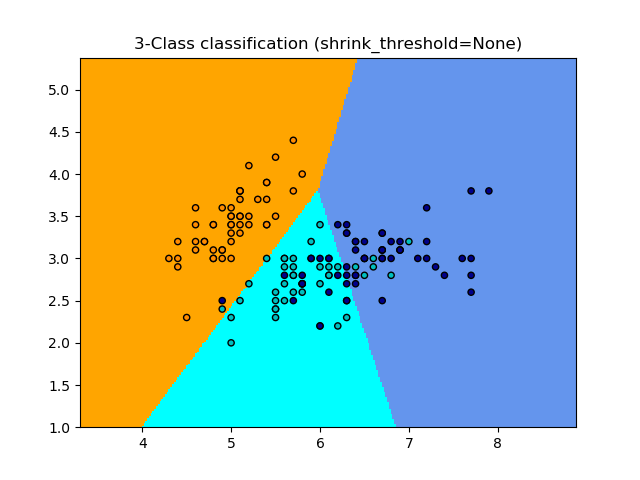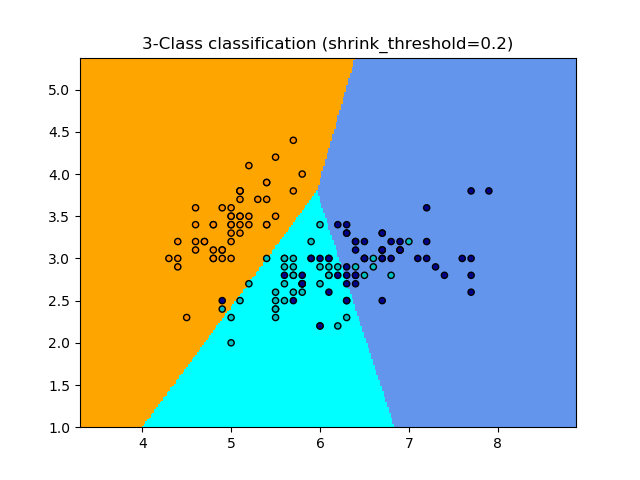# Nearest Centroid Classification¶

Sample usage of Nearest Centroid classification. It will plot the decision boundaries for each class.

••Out:

None 0.8133333333333334
0.2 0.82


print(__doc__)

import numpy as np
import matplotlib.pyplot as plt
from matplotlib.colors import ListedColormap
from sklearn import datasets
from sklearn.neighbors import NearestCentroid

n_neighbors = 15

# import some data to play with
# we only take the first two features. We could avoid this ugly
# slicing by using a two-dim dataset
X = iris.data[:, :2]
y = iris.target

h = .02  # step size in the mesh

# Create color maps
cmap_light = ListedColormap(['orange', 'cyan', 'cornflowerblue'])
cmap_bold = ListedColormap(['darkorange', 'c', 'darkblue'])

for shrinkage in [None, .2]:
# we create an instance of Neighbours Classifier and fit the data.
clf = NearestCentroid(shrink_threshold=shrinkage)
clf.fit(X, y)
y_pred = clf.predict(X)
print(shrinkage, np.mean(y == y_pred))
# Plot the decision boundary. For that, we will assign a color to each
# point in the mesh [x_min, x_max]x[y_min, y_max].
x_min, x_max = X[:, 0].min() - 1, X[:, 0].max() + 1
y_min, y_max = X[:, 1].min() - 1, X[:, 1].max() + 1
xx, yy = np.meshgrid(np.arange(x_min, x_max, h),
np.arange(y_min, y_max, h))
Z = clf.predict(np.c_[xx.ravel(), yy.ravel()])

# Put the result into a color plot
Z = Z.reshape(xx.shape)
plt.figure()
plt.pcolormesh(xx, yy, Z, cmap=cmap_light)

# Plot also the training points
plt.scatter(X[:, 0], X[:, 1], c=y, cmap=cmap_bold,
edgecolor='k', s=20)
plt.title("3-Class classification (shrink_threshold=%r)"
% shrinkage)
plt.axis('tight')

plt.show()


Total running time of the script: ( 0 minutes 0.690 seconds)

Estimated memory usage: 8 MB

Gallery generated by Sphinx-Gallery